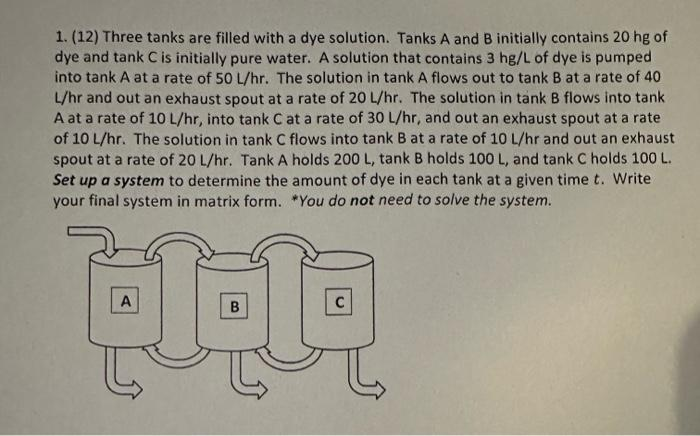Home / Expert Answers / Calculus / differential-equation-laplace-translation-theorems-1-12-three-tanks-are-filled-with-a-dye-solut-pa194

# (Solved): Differential Equation: Laplace Translation Theorems 1. (12) Three tanks are filled with a dye solut ...

Differential Equation: Laplace Translation Theorems1. (12) Three tanks are filled with a dye solution. Tanks and B initially contains of dye and tank is initially pure water. A solution that contains of dye is pumped into tank at a rate of . The solution in tank flows out to tank at a rate of 40 and out an exhaust spout at a rate of . The solution in tank B flows into tank A at a rate of , into tank at a rate of , and out an exhaust spout at a rate of . The solution in tank flows into at a rate of and out an exhaust spout at a rate of . Tank holds , tank holds , and tank holds . Set up a system to determine the amount of dye in each tank at a given time . Write your final system in matrix form. "You do not need to solve the system.

We have an Answer from Expert

Given data:

Three tanks are filled with a dye solution. Tanks   and   initially contains   hg of dye and tank   is initially pure water. A solution that contains   of dye is pumped into tank   at a rate of    The solution in tank   flows out to tank   at a rate of   and out an exhaust spout at a rate of   . The solution in tank    flows into tank    at a rate of   , into tank   at a rate of   , and out an exhaust spout at a rate of

To find:

Set up a system to determine the amount of dye in each tank at a given time   .
Write your final system in matrix form.

Now,

Let    denote the amounts (in hg) of dye in tanks   and   , respectively.